#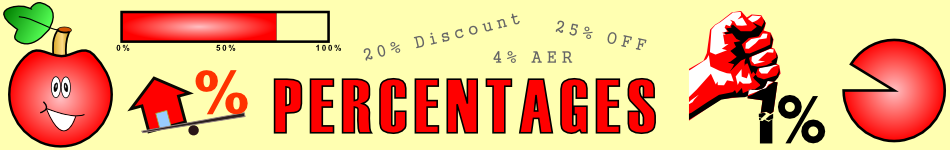There are 366 different Starters of The Day, many to choose from. You will find below some starters on the topic of Percentages. A lesson starter does not have to be on the same topic as the main part of the lesson or the topic of the previous lesson. It is often very useful to revise or explore other concepts by using a starter based on a totally different area of Mathematics.

Main Page

### Percentages Starters:Estimate the percentages of full circles and rectangles the sectors represent.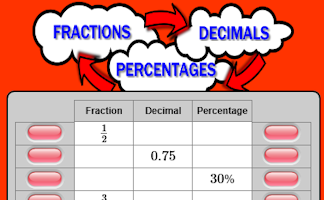Convert fractions to decimals, decimals to percentages and percentages to fractions.Finding a good personal loan requires an ability to calculate percentage and this page provides some practice.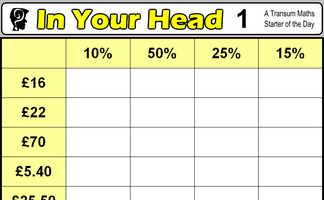Here are the simple percentage calculations everyone should be able to do in their heads.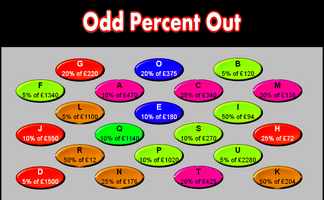A number of simple percentage calculations are given. The answers are in pairs. Which is the odd one out?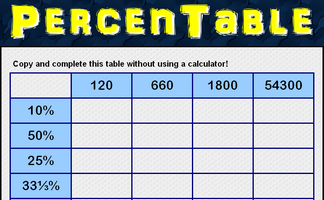Complete the table by calculating common percentages without using a calculator.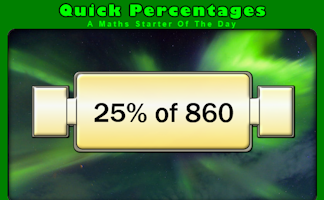Simple percentage questions appear on screen then fade every 8 seconds. This Starter is customisable.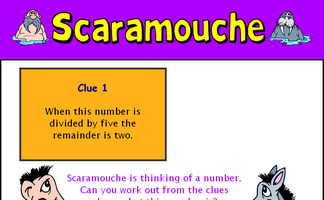Can you work out from the five clues given what the mystery number is?Work out which is the best scheme for Sid to choose for his summer bonus. One scheme involves a common misconception about percentages.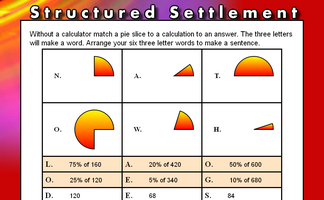Without a calculator match a a pie slice to a calculation to an answer.

## Exercises#### Compound Interest Calculator

A customised online calculator for quickly finding the solutions to compound interest problems.#### Estimating Percentages

Estimate the percentages represented by the diagrams.#### Express as a Percentage

This self-marking quiz requires you to work out what one quantity is as a percentage of a second quantity.#### Fraction Percentage

Match the fraction with the equivalent percentage. A drag and drop self marking exercise.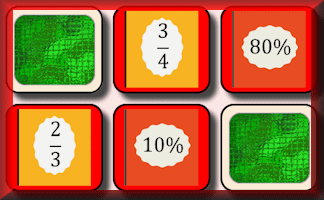#### Fraction Percentage Pairs

The traditional pairs or Pelmanism game adapted to test knowledge of simple fractions and their equivalent percentages.#### Fractions Decimals Percentages

Revise the methods for converting fractions to decimals and percentages.#### Fractions, Decimals, Percentages

An exercise on converting fractions to decimals, decimals to percentages and percentages to fractions.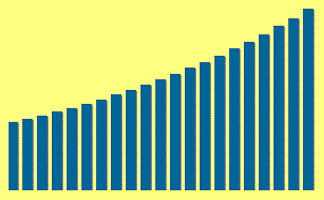#### Interest

Practise using the formulas for simple interest and compound interest.#### Particular Pipes

Construct the pipes using a set number of pieces with lengths given as fractions, decimals or percentages.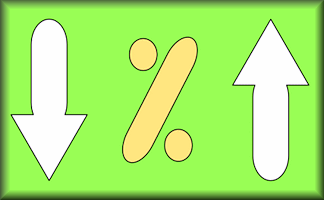#### Percentage Change

Test your understanding of using percentages with this self marking quiz about percentage change.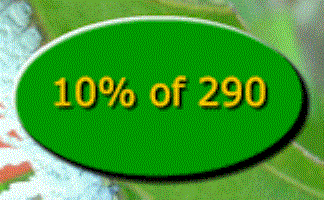#### Percentages

Practise calculating simple percentages in your head with this animated visual aid.

### Search

The activity you are looking for may have been classified in a different way from the way you were expecting. You can search the whole of Transum Maths by using the box below.

Have today's Starter of the Day as your default homepage. Copy the URL below then select
Tools > Internet Options (Internet Explorer) then paste the URL into the homepage field.

Set as your homepage (if you are using Internet Explorer)

Do you have any comments? It is always useful to receive feedback and helps make this free resource even more useful for those learning Mathematics anywhere in the world. Click here to enter your comments.For All: